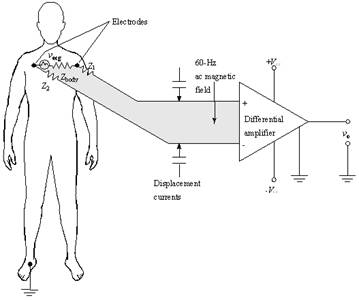## High Bandwidth Low Noise Amplifier with improved stability over Radio Frequency Range

Abstract

The measuring appliance amplifier is a double-end input differentiator amplifier. This amplifier is very accurate and highly stable. Its output zero reference voltage establishes willfully through the REF base pin’s voltage.

The AD620 input end will reach as high as 109O the input impedance. Its output offset voltage is 400uV and Input offset voltage is 30uV. The input bias current is very low, it is highest in 0.5nA and will not cross 2nA. When the gain is 100, gain error will be 0.15%. It increases 100:00 syntype rejection ratios to reach high i.e. 130dB. 9nV/ is Input noise and 72nV/ is the output noise.  The AD620 temperature stability is also required. The input offset voltage average temperature coefficient is 0.3uV/ and output offset voltage average temperature coefficient is 5.0uV/.Fig: The AD620 finds its major usage in ECG systems

Here, we shall explore the internal working of the AD620 and try to emulate the characteristics of AD620.

1) In this two-stage op-amp circuit, the resistor R is the only passive element. Biasing (i.e. setting up a constant dc bias current Ib) is the main function of the Resistor R. Later, this current is replicated at various other locations for biasing other amplifier stages via current mirrors. The bias current Ib is the input current for the current mirror in the op-amp circuit. N0 and N1 are two outputs.

2) N2 and P2 are combined together to form current mirrors and they distributes Ib to the rest circuit. Here, the input side of the mirror is N0 with two outputs of the mirror. Here, N3 and P3 will share the same gate-source voltage. So, the driver source N0 can replicate the bias current Ib as needed for biasing throughout the rest of the circuit. By using different aspect ratios W/L of the mirror output transistors with respect to the input transistor, we can scale the bias currents as required.

3) N1 and N0 are combined together to form the input differential pair. It is also the input of the first gain stage. The current mirrors for the differential pair N0 and N1 are P0 and P1. The voltage source VDD provides the bias current. It also works as a DC current source.

4) The common source gain stage is M6 and it is the second gain stage. Note that the input of this gain stage is at node 1 and Node 1 is the gate of P3. The output of this stage is at node 2 and Node 2 is the drain of M6. M6 is biased from node 1. The DC supply voltage VDD is directly connected to the source of M6. Hence, there is no signal component.

🔥95 Views

### Bhuvish

This site uses Akismet to reduce spam. Learn how your comment data is processed.